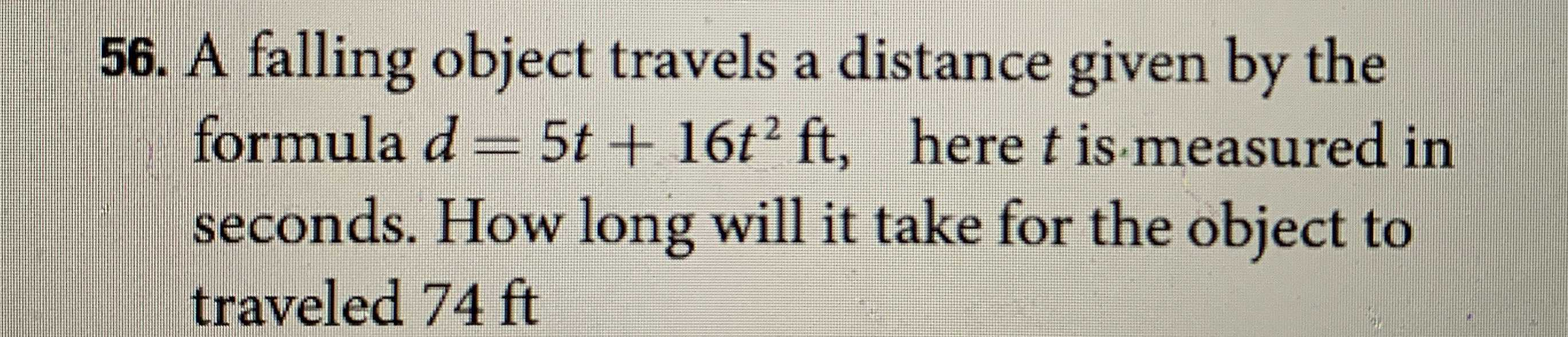### ¿Todavía tienes preguntas de matemáticas?

Pregunte a nuestros tutores expertos
Algebra
PreguntaA falling object travels a distance given by the formula $$d = 5 t + 16 t ^ { 2 } ft$$ , here $$t$$ is measured in seconds. How long will it take for the object to traveled $$74 ft$$

5t+16t$$^{2}$$=74
16t$$^{2}$$+5t-74=0